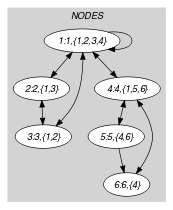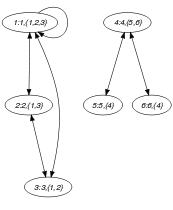## 5.394. symmetric

Origin
Constraint

$\mathrm{𝚜𝚢𝚖𝚖𝚎𝚝𝚛𝚒𝚌}\left(\mathrm{𝙽𝙾𝙳𝙴𝚂}\right)$

Argument
 $\mathrm{𝙽𝙾𝙳𝙴𝚂}$ $\mathrm{𝚌𝚘𝚕𝚕𝚎𝚌𝚝𝚒𝚘𝚗}\left(\mathrm{𝚒𝚗𝚍𝚎𝚡}-\mathrm{𝚒𝚗𝚝},\mathrm{𝚜𝚞𝚌𝚌}-\mathrm{𝚜𝚟𝚊𝚛}\right)$
Restrictions
 $\mathrm{𝚛𝚎𝚚𝚞𝚒𝚛𝚎𝚍}$$\left(\mathrm{𝙽𝙾𝙳𝙴𝚂},\left[\mathrm{𝚒𝚗𝚍𝚎𝚡},\mathrm{𝚜𝚞𝚌𝚌}\right]\right)$ $\mathrm{𝙽𝙾𝙳𝙴𝚂}.\mathrm{𝚒𝚗𝚍𝚎𝚡}\ge 1$ $\mathrm{𝙽𝙾𝙳𝙴𝚂}.\mathrm{𝚒𝚗𝚍𝚎𝚡}\le |\mathrm{𝙽𝙾𝙳𝙴𝚂}|$ $\mathrm{𝚍𝚒𝚜𝚝𝚒𝚗𝚌𝚝}$$\left(\mathrm{𝙽𝙾𝙳𝙴𝚂},\mathrm{𝚒𝚗𝚍𝚎𝚡}\right)$
Purpose

Consider a digraph $G$ described by the $\mathrm{𝙽𝙾𝙳𝙴𝚂}$ collection. Select a subset of arcs of $G$ so that the corresponding graph is symmetric (i.e., if there is an arc from $i$ to $j$, there is also an arc from $j$ to $i$).

Example
$\left(\begin{array}{c}〈\begin{array}{cc}\mathrm{𝚒𝚗𝚍𝚎𝚡}-1\hfill & \mathrm{𝚜𝚞𝚌𝚌}-\left\{1,2,3\right\},\hfill \\ \mathrm{𝚒𝚗𝚍𝚎𝚡}-2\hfill & \mathrm{𝚜𝚞𝚌𝚌}-\left\{1,3\right\},\hfill \\ \mathrm{𝚒𝚗𝚍𝚎𝚡}-3\hfill & \mathrm{𝚜𝚞𝚌𝚌}-\left\{1,2\right\},\hfill \\ \mathrm{𝚒𝚗𝚍𝚎𝚡}-4\hfill & \mathrm{𝚜𝚞𝚌𝚌}-\left\{5,6\right\},\hfill \\ \mathrm{𝚒𝚗𝚍𝚎𝚡}-5\hfill & \mathrm{𝚜𝚞𝚌𝚌}-\left\{4\right\},\hfill \\ \mathrm{𝚒𝚗𝚍𝚎𝚡}-6\hfill & \mathrm{𝚜𝚞𝚌𝚌}-\left\{4\right\}\hfill \end{array}〉\hfill \end{array}\right)$

The $\mathrm{𝚜𝚢𝚖𝚖𝚎𝚝𝚛𝚒𝚌}$ constraint holds since the $\mathrm{𝙽𝙾𝙳𝙴𝚂}$ collection depicts a symmetric graph.

Typical
$|\mathrm{𝙽𝙾𝙳𝙴𝚂}|>2$
Symmetry

Items of $\mathrm{𝙽𝙾𝙳𝙴𝚂}$ are permutable.

Algorithm

The filtering algorithm for the $\mathrm{𝚜𝚢𝚖𝚖𝚎𝚝𝚛𝚒𝚌}$ constraint is given in [Dooms06]. It removes (respectively imposes) the arcs $\left(i,j\right)$ for which the arc $\left(j,i\right)$ is not present (respectively is present). It has an overall complexity of $O\left(n+m\right)$ where $n$ and $m$ respectively denote the number of vertices and the number of arcs of the initial graph.

Keywords
Arc input(s)

$\mathrm{𝙽𝙾𝙳𝙴𝚂}$

Arc generator
$\mathrm{𝐶𝐿𝐼𝑄𝑈𝐸}$$↦\mathrm{𝚌𝚘𝚕𝚕𝚎𝚌𝚝𝚒𝚘𝚗}\left(\mathrm{𝚗𝚘𝚍𝚎𝚜}\mathtt{1},\mathrm{𝚗𝚘𝚍𝚎𝚜}\mathtt{2}\right)$

Arc arity
Arc constraint(s)
$\mathrm{𝚒𝚗}_\mathrm{𝚜𝚎𝚝}$$\left(\mathrm{𝚗𝚘𝚍𝚎𝚜}\mathtt{2}.\mathrm{𝚒𝚗𝚍𝚎𝚡},\mathrm{𝚗𝚘𝚍𝚎𝚜}\mathtt{1}.\mathrm{𝚜𝚞𝚌𝚌}\right)$
Graph class
$\mathrm{𝚂𝚈𝙼𝙼𝙴𝚃𝚁𝙸𝙲}$

Graph model

Part (A) of Figure 5.394.1 shows the initial graph from which we start. It is derived from the set associated with each vertex. Each set describes the potential values of the $\mathrm{𝚜𝚞𝚌𝚌}$ attribute of a given vertex. Part (B) of Figure 5.394.1 gives the final graph associated with the Example slot.

##### Figure 5.394.1. Initial and final graph of the $\mathrm{𝚜𝚢𝚖𝚖𝚎𝚝𝚛𝚒𝚌}$ set constraint(a) (b)